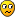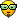## Why the spiral vortex is a natural phenomenom

Beyond the boundaries of established science an avalanche of exotic ideas compete for our attention. Experts tell us that these ideas should not be permitted to take up the time of working scientists, and for the most part they are surely correct. But what about the gems in the rubble pile? By what ground-rules might we bring extraordinary new possibilities to light?

Moderators: bboyer, MGmirkin

StevenO
Posts: 894
Joined: Tue Apr 01, 2008 11:08 pm

### Why the spiral vortex is a natural phenomenom

When attempting to discuss general solutions for Einsteins General Relativity on a Physics forum, I found the following identity:
• Momentum(Mass 1) + Momentum(Mass 2) = Mass 1 * Mass 2 * c^4
For simplicity, assume Mass 1 = Mass 2, then we get:
• Mass * Velocity 1 + Mass * Velocity 2 = Mass^2 * c^4,

or
• Velocity 1 + Velocity 2 = Mass * c^4
So what? Well ...

if one realizes that velocities are vectors, you see that the velocity vectors of two bodies cannot be exactly opposite!
If that happens a torque will be generated that is proportional to the Mass.

As a result the two bodies will create a double helix movement when the momentum vectors point into eachother.

Anybody thinks this is crazy? According to the physics forum it is nonsense....
First, God decided he was lonely. Then it got out of hand. Now we have this mess called life...
The past is out of date. Start living your future. Align with your dreams. Now execute.

junglelord
Posts: 3693
Joined: Mon Mar 17, 2008 5:39 am

### Re: Why the spiral vortex is a natural phenomenom

The more I learn APM and think in those terms, the data from the standard model is good, the theory is crap.
If one does not have proper definitions for dimensions, units, constants, measurements and does not build in dimensions with geometry, its all mixed up. I have given up trying to understand things with the standard model.

Therefore what is mass?If your definiton of mass is a dimension, which it is not, then that makes no sense at all. Since mass is a single dimension and is not distributed, I get nothing from it.

The algebra of APM with quantum constants built of quantum units from quantum dimensions is fully supported that the aether field is full of innumerable vortex's. Since it is the primary archetype, then it will appear in all systems at some level.
If you only knew the magnificence of the 3, 6 and 9, then you would have a key to the universe.
— Nikola Tesla
Casting Out the Nines from PHI into Indigs reveals the Cosmic Harmonic Code.
— Junglelord.
Knowledge is Structured in Consciouness. Structure and Function Cannot Be Seperated.
— Junglelord

StevenO
Posts: 894
Joined: Tue Apr 01, 2008 11:08 pm

### Re: Why the spiral vortex is a natural phenomenom

junglelord wrote:The more I learn APM and think in those terms, the data from the standard model is good, the theory is crap.
If one does not have proper definitions for dimensions, units, constants, measurements and does not build in dimensions with geometry, its all mixed up. I have given up trying to understand things with the standard model.
Agreed. The observations are correct, but the explanations are a skyscraper of epicycles. Not sure if I agree on the dimensions and constants, since they are all relative. I think the only constants we need to explain our universe are e, pi and alpha (plus everything else we know from mathematics).
junglelord wrote:Therefore what is mass?If your definiton of mass is a dimension, which it is not, then that makes no sense at all. Since mass is a single dimension and is not distributed, I get nothing from it.
For sure my definition is not a dimension. It depends whether one interprets "mass" as an equivalent for energy or restrict it to (3D) matter. Energy is basically movement in the time domain, which can be in one dimension (heat, moment, torque, enthalphy), 2 dimensions (momentum, impulse, magnetic charge/flux, resistivity) or 3 dimensions (atom mass, inductance).
junglelord wrote:The algebra of APM with quantum constants built of quantum units from quantum dimensions is fully supported that the aether field is full of innumerable vortex's. Since it is the primary archetype, then it will appear in all systems at some level.
I take it that you would agree with the formula then. How does APM calculate these vortices?

If the formula is correct (I have not been able to disprove it yet) then I think it can explain a lot of things, a.o.:

- differences between elastic and non-elastic collisions
- differences between laminar/turbulent fluid flow
- pair production
- dense plasma focus
- gamma ray bursts
- stellar explosions/quasars
- flux ropes
- magnetic reconnection
- eddy currents
- plasma pinch
- sunspots
- ...
First, God decided he was lonely. Then it got out of hand. Now we have this mess called life...
The past is out of date. Start living your future. Align with your dreams. Now execute.

junglelord
Posts: 3693
Joined: Mon Mar 17, 2008 5:39 am

### Re: Why the spiral vortex is a natural phenomenom

I can send you an email with a link. I am writing a detailed question and answer for Secrets of the Aether. You can observe the way APM calculates everything with simple algebra.APM data collects in dimensional and geometric form, processes in dimensional and geometric form, which materializes equations in dimensional and geometric form. By keeping dimensional and geometric form with data and equations, old concepts die, for example, the Aether unit and its geometry, will influence the understanding of physics.
All quantum units are also quantum constants. This is possible because the universe arose from very precise, first measurements. A quantum measurements apply equally to force, matter, and the environment, as they all arise from the same source. A physics system were quantum units are also quantum constants has many advantages, particularly when we examine the qualities of atoms and their interactions.

Why should the measurements be quantum at all? If the quantum measurements of atoms did not exist, there would be no conservation laws and universe would lack a reliable framework. It is because there is a single either unit, a single value for electron mass, a single value for proton mass, a single quantum length, a single quantum frequency, a single quantum electrostatic charge, a single quantum strong charge of the electron, and a single quantum strong charge of the proton, that we can make predictions about the universe at all.

At the level of quantum existence, each interaction will be the same on earth as in some distant galaxy. This means not only will the electron angular momentum be the same in all places and at all times, but also that the velocity of light in a vacuum, the permeability, the conductance, and the permittivity of aether will be the same.
Secrets of the Aether, chapter 7, Constants, page 151
The actual aether is all vortex's. Its due to the fundamental dimensions (length, frequency, mass, charge, spherical geometry) structural relationships. Include that information along with primary angular momentum (Plancks Constant) and the two constants, pi, PHI-phi, that the geometry of the quantum world is also vortex. The aether structure and the quantum structure is simple in algebraic form in APM. Its all very equal and understandable. Remember that the vortex makes all other geometric forms. Add that concept to the information on the Zome video "2,3,5 Infinity" and voila, we have Sacred Geometry as an expression of the vortex. Of course Zome is a wonderful tool to understand the geometry of the atomic molecular world and also dimensions above four and below three and how things are embedded like the vortex is embedded in everything.How does the structure of the non material dimensions make vortex's? Mass being a primary dimension, of no length, but a function of PHI, is therefore a spiral. That is just one example. Mass does not ever change. It is not equal to matter and does not convert to energy. Energy is a unit of three dimensions. Mass is a dimension. Fundamental quantum dimensions, build into quantum units, which are quantum constants. Fundamental dimensions do not convert into anything. They remain what they are, fundamental dimensions. Thats why the spiral vortex is the archetype geometric structure, due to the non material aether structure which creates all matter from five fundamental dimensions with angular momentum, via three forces and the Gforce.
28. Does E = mc squared qualify in APM?
No

29. Why does E = mc squared, not qualify in APM?
Because the variables have dimensions but no value. Therefore it is not a true equation, rather an expression or formula.

If E and m had inherent value, as does the constant c then E = 1 unit and m = 1 unit
E = joule
m = kg

E = mc^2
Joule = kg X 8.988 X10^16 (m^2/sec^2)

through transposition this would resolve as
1 = 8.988 x 10^16

Therefore E does not equal mc squared because there is no true equality! If you apply a constant to both E and m things are no where near equal!

Energy is a unit, mass is a dimension
E is a unit composed of the dimensions of mass, length, frequency.
E = M X L^2 X F^2

When it comes down to the truth to the truth of it, mass is a dimension while energy is a unit made up of three dimensions.

In SR, mass converts to energy as an object approaches the speed of light. If this were true, then instead of having an infinite mass as the theory proposes, a spaceship should be massless at the speed of light. However, if the spaceship were massless at the speed of light, then it would have no energy because the mass is zero.. No double the die hard relativists will come forth with arguments that there is relativistic mass, which is different from rest mass.

Mass is ultimately only a dimension. Mass is not equal to matter or energy. Mass does not rest and does not move as an independent entity. There is no such "thing" as mass that can be converted to energy, of which energy is merely a unit.
If you only knew the magnificence of the 3, 6 and 9, then you would have a key to the universe.
— Nikola Tesla
Casting Out the Nines from PHI into Indigs reveals the Cosmic Harmonic Code.
— Junglelord.
Knowledge is Structured in Consciouness. Structure and Function Cannot Be Seperated.
— Junglelord

lizzie
Guest

### Re: Why the spiral vortex is a natural phenomenom

Junglelord saqid:
The algebra of APM with quantum constants built of quantum units from quantum dimensions is fully supported that the aether field is full of innumerable vortex's.
Since it is the primary archetype, then it will appear in all systems at some level.
http://wefooleffect.com/index.htm

### Who is online

Users browsing this forum: No registered users and 4 guests